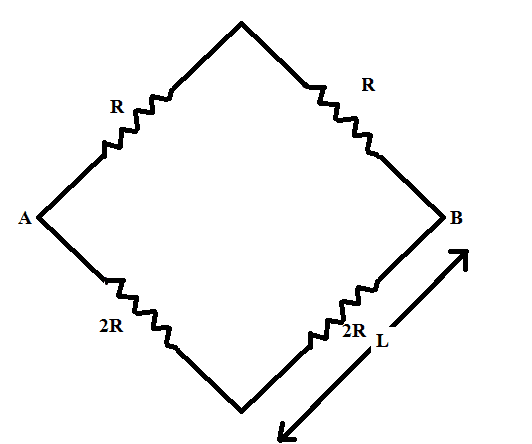# Calculation of magnetic field

## Homework Statement[/B]
Consider a square whose successive sides of length $L$ has resistances $R, 2R, 2R, R$ respectively. If a potential difference $V$ is applied between the points (call them , say , A and B) where the sides with R and 2R meet. Find the magnetic field $B$ at the center of the square.

## Homework Equations

$R_s = R_1 + R_2 + R_3 + ... + R_n$
$\frac{1}{R_p} = \frac{1}{R_1} + \frac{1}{R_2} + \frac{1}{R_3} + ... + \frac{1}{R_n}$
$V = iR$
$\oint \vec B \cdot d \vec s = \mu _0 i$

## The Attempt at a Solution

The equivalent resistance of this combination is $\frac{4R}{3}$ . So, the current through $R , 2R$ are $\frac{V}{2R} , \frac{V}{4R}$ respectively. But, to calculate the magnetic field, what will be the amperian loop to imagine?

TSny
Homework Helper
Gold Member
Does the magnetic field pattern have enough symmetry to use Ampere's law? If not, can you think of a different law that you can use?

So, I should use Biot-Savart's law. But another question, can I use Biot-Savart's Law in case of variable electric field?

BvU
•